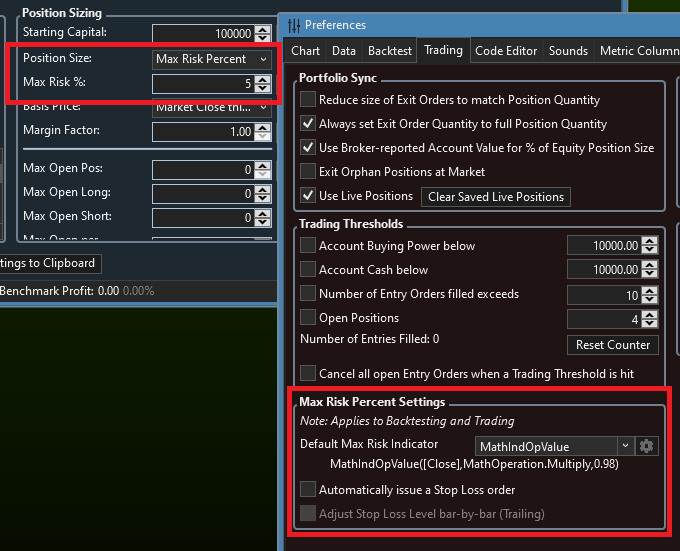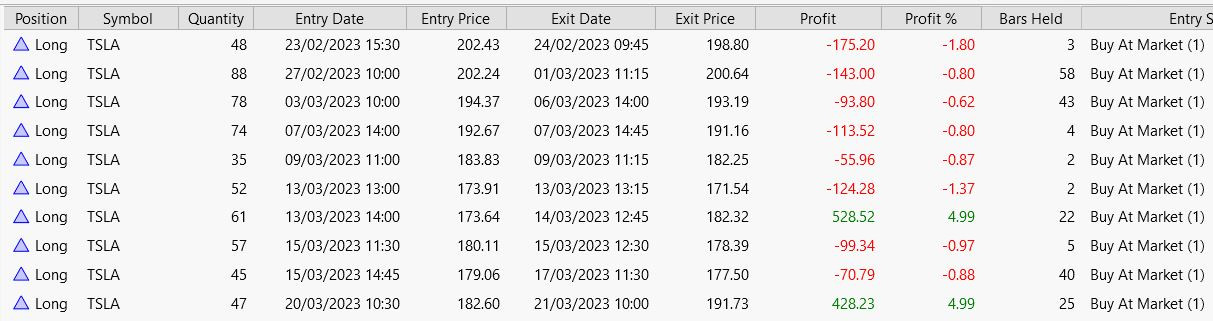Hi, is there any way to perform the following calculation for position sizing via the Position Size Andvanced function or only possible with custom C# coding?

Assumption account size 100,000
Assumption stock entry price \$120
Maximum risk for a position 5% of the account size (here \$500)
StopLoss should be 2% below the entry price (\$117.60)

Calculation example:
Risk 5% account size / (entry price \$120 - StopLoss price \$117,60)

Result 208.33 with rounding down 208.

LG Christian
0
322
Solved
16 Replies

Bookmark

Sort
You're looking for the Max Risk Percent Position Sizing option (WL8 B11+):

Build Highlights Video: https://youtu.be/xkzJZuYei_o
0
That's the Max Risk % sizing, and although you can't specify the entry price without coding it, you can use 2% below the last Close (as shown in the image) - or any other indicator that you can configure in the preferences.By the way, you can't really use the entry price to calculate sizing because the sizing has to take place before you enter the position. You have to know the "MaxRiskStopLevel" when you Place the trade (the intended stop level). That's what you're specifying with the indicator shown above. Later, in code, you can adjust the actual stop loss based on the execution price.
0
I watched the video and I understand the Stop Loss calculation.

I don't understand how WealthLab is calculating what is the Position Size if there are 3 concurrent signals on a \$100,000 account. and a Stop loss of 10%.

If I use %of equity, I could say Position size is 33% for each asset.

However, what is the calculation for Position Size for MaxRisk % of 10% when there are 3 assets. Is it assuming I am trading the full account size or less for each asset?

So for my above example if the stock price is \$100 for asset A, \$200 for asset B and \$300 for asset C, what is the initial position size for each trade. I understand the stop loss would be 10% for each trade.

To me, the stop loss says place a limit order, so that if each stock price goes down by 10% exit.

However, how does Wealthlab tell me how many shares to buy for each asset.

Thank you,
Larry
0
Hi Larry. It's standard Max Risk % Sizing. It has nothing to do with splitting equity equally between positions. It's a TOTALLY different concept that attempts to keep the maximum loss of every trade to a percentage of the equity. (10% would be far too high a number to use.)

It goes like this (assuming stocks):

1. You set what percentage of your equity to risk for each trade.
(Edit: the percentage is the amount of your account value that you're willing to lose. So, 1% Max Risk Sizing for a \$50K account means that you intend not to lose more than \$500 per trade.)

2. You set a stop price. You should make the strategy to take a loss at that price, but it's not required. The price is set in either of 2 ways:
a. Preferences > Trading > Max Risk Percent Settings > Default Max Risk Indicator
b. In C# Code by overriding GetMaxRiskStopLevel(). If you use this, the other is ignored.

3. How is Max Risk % Sizing Calculated?
Simple - it's the number of shares to buy at the current price that will lose the specified % of equity value at the max risk stop price.

Example:
Assume \$50K equity, 3% Max Risk. Say you buy AAPL at \$150 LIMIT and your stop price is \$142. The number of shares, then, is:

\$ Risk = \$50000 * 3% = \$1500
Shares = \$1500 / (150 - 142) = 187. 5 => 187 shares

In summary, if you truly follow the Max Risk % Sizing strategy, your trading strategy will buy 187 shares of AAPL at 150 and you control the risk by selling at a stop loss if price hits 142. If it goes there, 187 shares will lose about \$1500.

If price gaps lower than 142, you can lose more.
2
Cone,
I understand what you are saying.

My question, is how do I know how many shares to buy when there are more than 1 signal.

How does Wealthlab make this decision with the above 3 signal example for a single strategy.

Also, without programming, can I change these settings so that I only want to trade a portion of my account, such as % of equity.

My concern is I can make the 3 signals only loose 10% by trading 100% of the account, or much less such as 80% of the account. I can still control losses with a stop loss of 10%. So that if I trade 100% of the account, then the stop loss would be smaller on 10%, then if I traded 80% of the account.

To me, I understand how Wealthlab creates a stop loss and Max Risk on a single signal. I don't understand how it does this with 3 signals, because I want to know the position size of each asset and how it calculated that position size.

Thank you.,
Larry
0
Are you saying you’d like to adjust the position size based on the number of entry signals? You’d have to use an advanced position sizer for that, or it’s also possible to code that up in the PostExecute method, adjusting the Transaction quantities based on how many entry Transactions are in the Backtester.Orders.
0
Glitch,

I am trying to understand how many shares will be in a buy signal using the Position Size of max Risk Percent of 10% when the number of signals will vary when a strategy is run on a portfolio.

What I am saying is the portfolio universe is 737 stocks. The strategy returns a non fixed number of symbols. Strategy can return 0 signals, 1 or more signals, etc. I have a 100,000 starting capital and I choose Position Size = Max Risk Percent of 10%.

So if I run the strategy and 1 signal is returned:
How many shares are in the buy signal if strategy returns 1 signal to buy an asset priced at \$100.

How many shares are in each signal if strategy returns 2 signal to buy 2 assets when 1 asset is priced at \$100 and the other asset is priced at \$200.

Thank you,
Larry

0
The number of signals has no bearing on it.
0
Glitch,

Got it.

I was able to figure out by running a backtest and seeing results how I want to use these features.

I do see using the Advanced Risk Sizer, using max Risk % limited to % of Equity and configuring Position sizer giving me consistent control of understanding how much risk.

Very useful feature.

Larry
0
I am using GetMaxRiskStopLevel from this https://www.wealth-lab.com/Discussion/Max-risk-percent-position-sizing-in-WL7-8544 thread, but instead of 1.5 atr I use 1, and the same 1 atr for stop loss.

Max risk is set to 2%, it means every sl hit is -2% from the account. Starting capital 10k.

It turns out this way:Although according to the description the first trade should be strictly -200, the second a little less than -200, and so on progressively. Although here we see a very large dispersion. At least doubled the number of shares on the second trade.
0
Wouldn't you expect more shares for the same Max Risk % when ATR is smaller and fewer shares when it's larger? If not, why don't you expect that?
0
> If not, why don't you expect that?
Well, from it's description "Sizes such that if the trade stops out, it will result in a loss of the specified percentage". So I expected each stop to be exactly 2%, then the increase in the number of shares would make sense. But while writing this post I opened the help and there I noticed another text "This position sizing method you specify the maximum percentage loss you're willing to take on a trade". That's what misled me initially, I expected exact not maximum. In general I want to say that I do not understand how it works, but it seems that it works.
0
It works like this,

Say your MAX RISK is 10%, on an entry of share price \$100. Your stop loss is 20%, and you have \$100,000 equity.

If you buy 500 shares at \$100, and your stop loss of 20% is hit, then you wind up losing \$20 per share, or (20 * 500 = \$10,000) which is 10% of the \$100,000 equity.

So, in this case the appropriate position size would be 500 shares.
1
> It works like this,
Verified that on a simple strategy with a fixed percent stop on \$100 stock. Works exactly as described.
0
QUOTE:
If you buy 500 shares at \$100, and your stop loss of 20% is hit, then you wind up losing \$20 per share, or (20 * 500 = \$10,000) which is 10% of the \$100,000 equity.

So , it simply means that MAX RISK % is the risk of margin used to enter a particular position. Risk % from Margin , not from Balance.

Could you please describe the opportunity to use the Risk from Balance or Buying Power, not from Margin?
as WW5 said MAX RISK from Margin leads to kind of dispersion and results in absolutely different PnL report.
0
Guys, WealthLab didn't invent Max Risk % sizing. There is plenty of documentation all over the internet and trading books, like Van Tharp's "Trade Your Way to Financial Freedom".

It is not risk of margin. It is the percentage of account value that you WILL LOSE if you exit at the price you have defined as the initial stop. I gave a very specific example in Post #4 above.

I think the problem everyone is having is when we say "% of equity to risk". It's true that if you buy a stock like SIVB, SBNY, Enron, (or any other) all of your equity is at risk. But in the context of Max Risk %, we're saying this is the percentage of equity that we're willing to lose by stopping out. The loss can be much more if price gaps lower than your stop price.
0